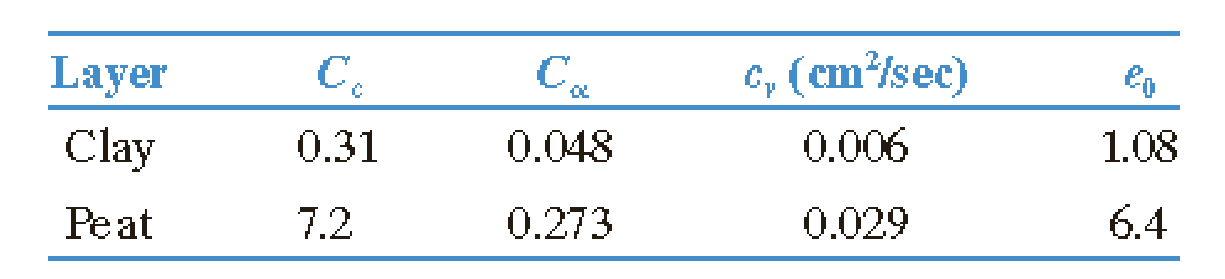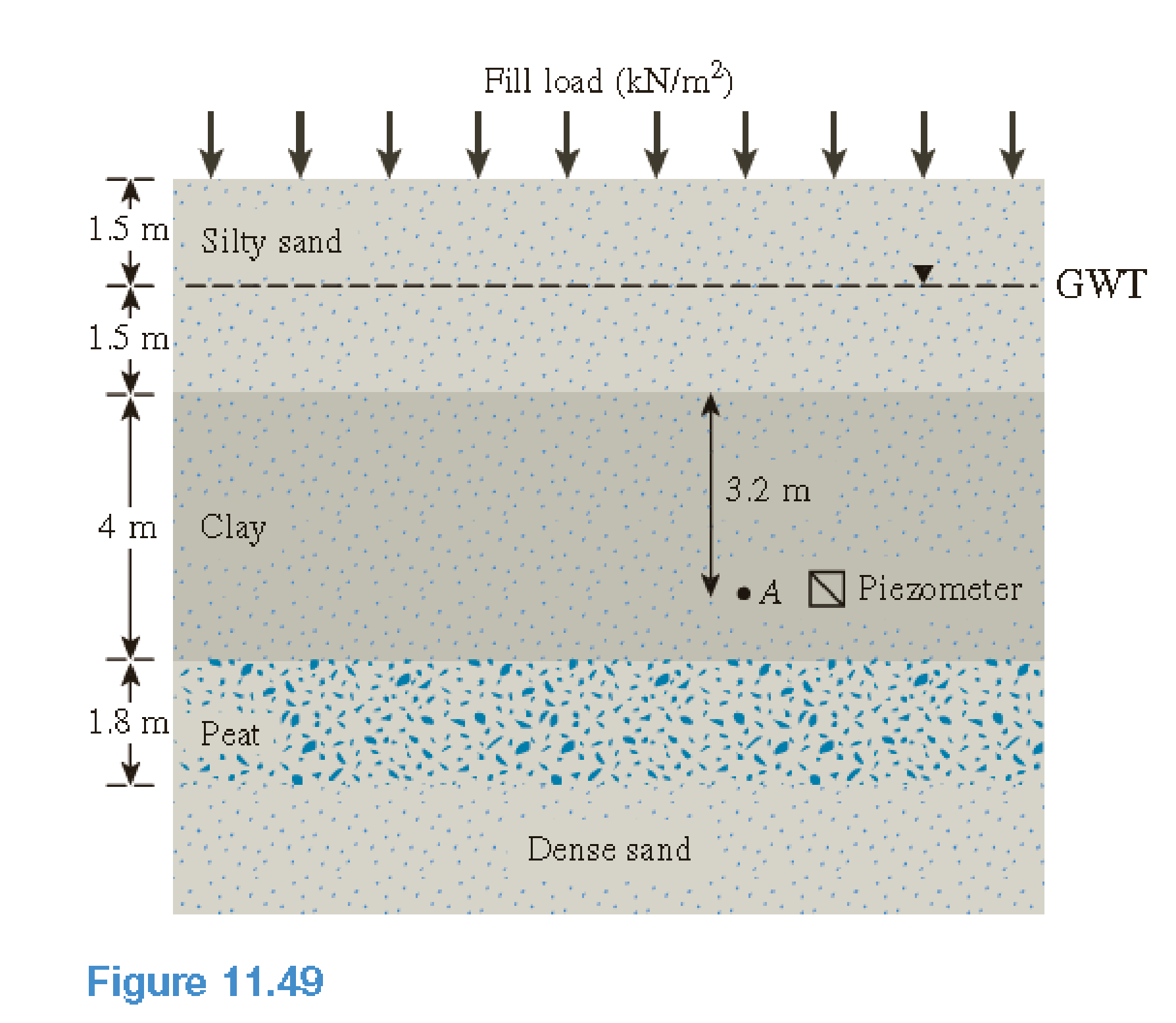Chapter 11, Problem 11.1CTPPrinciples of Geotechnical Enginee...

9th Edition
Braja M. Das + 1 other
ISBN: 9781305970939

Solutions

Chapter
SectionPrinciples of Geotechnical Enginee...

9th Edition
Braja M. Das + 1 other
ISBN: 9781305970939
Textbook Problem

Foundation engineers are often challenged by the existence of soft compressible soils at the construction site. Figure 11.49 shows a soil profile with a silty sand ( γ = 17   kN / m 3 ;   γ sat = 19.2   kN / m 3 ) underlain by high plasticity clay ( γ sat = 18.8   kN / m 3 ) and a peat layer ( γ sat = 15   kN / m 3 ) , followed by dense sand. To expedite consolidation and minimize future settlement, an additional 1.75-m thick fill material, compacted to a unit weight of 20.1 kN/m3, will be placed on top of the silty sand layer. The plan area of the fill is 8 m × 8 m. The fill load will be left in place for 18 months, after which construction will begin with the fill becoming part of the permanent foundation. Undisturbed samples collected from the clay and organic layers had the following properties:a. Estimate the total consolidation settlement under the action of the fill load. Consider both the clay and peat layers to be normally consolidated. b. Estimate the time for 99% primary consolidation in each layer. Are the layers singly or doubly drained? Explain. c. Estimate the secondary compression in each layer up to end of 18 months. d. What will be the total settlement after 18 months? e. What is the remaining excess pore water pressure at point A two months after the application of the fill load?f. Determine the effective stress at point A two months after the application of the fill load. g. A piezometer was installed at point A to monitor the pore water pressure. What should be the piezometer reading (upiezometer) two months after the fill load was applied?

(a)

To determine

Calculate the total consolidation settlement under the action of fill load.

Explanation

Given information:

The thickness of fill material (t) is 1.75m.

The compacted unit weight of fill material (γfill) is 20.1kN/m3.

The length of the foundation (L) is 8m.

The breadth of the foundation (B) is 8m.

The depth of fill (Df) is 1.5m.

The height of the layer silty sand (H1) is 3m.

The height of the clay layer (H2) is 4m.

The height of the peat layer (H3) is 1.8m.

The dry unit weight of sand (γd) is 17kN/m3.

The saturated unit weight of sand (γsat)sand is 19.2kN/m3.

The saturated unit weight of clay (γsat)clay is 18.8kN/m3.

The saturated unit weight of peat (γsat)peat is 15kN/m3.

The time (t) is 8months.

The properties of clay and organic layers are given in the Table.

Calculation:

Consider the unit weight of water (γw) is 9.81kN/m3.

Calculate the distributed load (q) as shown below.

q=tγfill

Substitute 1.75m for t and 20.1kN/m3 for γfill.

q=1.75×20.1=35.02kN/m2

Calculate the increase in vertical stress (Δσz) below the center of a rectangular area using the relation as follows.

Δσz=qI4 (1)

Here, I4 is the influence factor and as a function of m1 and n1.

For clay layer:

For the depth (z) of 3m:

Calculate the width (b) as shown below.

b=B2

Substitute 8m for B.

b=82=4m

Calculate the ratio (m1) as shown below.

m1=LB

Substitute 8m for L and 8m for B.

m1=88=1

Calculate the ratio (n1) as shown below.

n1=zb

Substitute 4m for b and 3m for z.

n1=34=0.75

Similarly calculate the remaining values and tabulate as in Table 1.

Refer Table 10.11 “Variation of I4 with m1 and n1” in the Text Book.

Take the value of I4 as 0.892, for the values m1 of 1 and n1 of 0.6.

Take the value of I4 as 0.800, for the values m1 of 1 and n1 of 0.8.

Calculate the value of I4 for the values m1 of 1 and n1 of 0.75 by interpolation as shown below.

0.80.750.80.6=0.8I40.80.8920.25×(0.092)=0.8I4I4=0.823

Similarly calculate the remaining values and tabulate as in Table 1.

Calculate the increase in vertical stress (Δσz) as shown below.

Substitute 35.02kN/m2 for q and 0.823 for I4 in Equation (1).

Δσz=35.02×0.823=28.82kN/m2

Similarly calculate the increase in vertical stress values and tabulate as in Table 1.

Show the increase in vertical stress for each depth below the center of the loaded area as in Table 1.

 m1=LB b=B2 Depth, z (m) n1=zb I4 Δσz (kN/m2) 1 4 3 0.75 0.823 28.82 1 4 5 1.25 0.599 20.98 1 4 7 1.75 0.403 14.11

Table 1

Refer to table 1.

Calculate the stress increase in the clay layer (Δσ) using the relation.

Δσ=Δσt+4Δσm+Δσb6 (2)

Here, Δσt is the increase in effective stress at the top layer, Δσm is the increase in effective stress at middle layer, and Δσb is the increase in effective stress at the bottom layer.

Substitute 28.82kN/m2 for Δσt, 20.98kN/m2 for Δσm, and 14.11kN/m2 for Δσb in Equation (2).

Δσ=28.82+4×20.98+14.116=126.856=21.14kN/m2

Calculate the average effective stress at the middle of the clay layer (σo) as shown below.

σo=γdDf+((γsat)sandγw)(H1Df)+((γsat)clayγw)×H22

Substitute 17kN/m3 for γd, 1.5m for Df, 19.2kN/m3 for (γsat)sand, 9.81kN/m3 for γw, 18.8kN/m3 for (γsat)clay, 3m for H1, and 4m for H2.

σo=17×1

(b)

To determine

Calculate the time for 99% primary consolidation for each layer.

(c)

To determine

Calculate the secondary compression in each layer up to end of 18 months.

(d)

To determine

Calculate the total settlement after 18 months.

(e)

To determine

Calculate the excess pore water pressure at point A two months after the application of the fill load.

(f)

To determine

Calculate the effective stress at point A two months after the application of the fill load.

(g)

To determine

Calculate the piezometer reading at point A two months after the application of the fill load.

Still sussing out bartleby?

Check out a sample textbook solution.

See a sample solution

The Solution to Your Study Problems

Bartleby provides explanations to thousands of textbook problems written by our experts, many with advanced degrees!

Get Started

Why is an object said to have greater semantic content than an entity?

Database Systems: Design, Implementation, & Management

Should Gladys call the legal authorities? Which agency should she call?

Principles of Information Security (MindTap Course List)

What are the potential costs of implementing a database system?

Database Systems: Design, Implementation, & Management

What type of fixed gage can be used to check a threaded hole?

Precision Machining Technology (MindTap Course List)

Identify how technology is used in the publishing industry.

Enhanced Discovering Computers 2017 (Shelly Cashman Series) (MindTap Course List)

How can a hose be checked for leaks?

Welding: Principles and Applications (MindTap Course List)